# Kinematic Equations NIS grade 11 physics reviewPage 2

#### WATCH ALL SLIDES

Motion is described by the equation d = vt

The slope (gradient) of the DT graph = Velocity

The steeper the line of a DT graph, the greater the velocity of the body

1

d(m)

2

3

v1 > v2 > v3

t(s)

Slide 10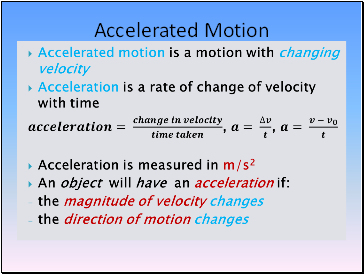## Accelerated Motion

Slide 11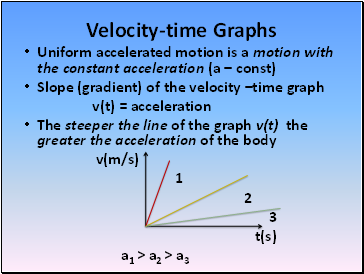## Velocity-time Graphs

Uniform accelerated motion is a motion with the constant acceleration (a – const)

Slope (gradient) of the velocity –time graph

v(t) = acceleration

The steeper the line of the graph v(t) the greater the acceleration of the body

v(m/s)

1

2

3

t(s)

a1 > a2 > a3

Slide 12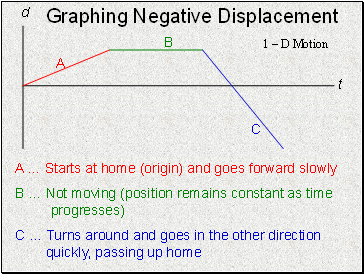## Graphing Negative Displacement

A … Starts at home (origin) and goes forward slowly

B … Not moving (position remains constant as time progresses)

C … Turns around and goes in the other direction quickly, passing up home

1 – D Motion

Slide 13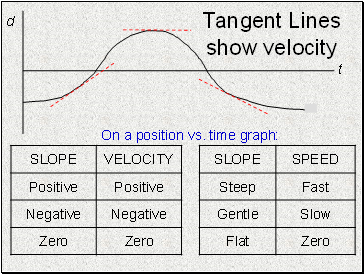Tangent Lines show velocity

t

d

On a position vs. time graph:

Slide 14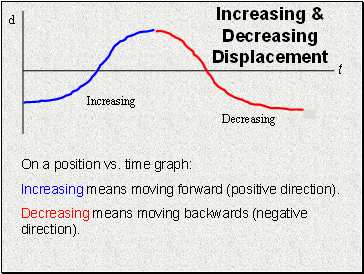Increasing & Decreasing Displacement

Increasing

Decreasing

On a position vs. time graph:

Increasing means moving forward (positive direction).

Decreasing means moving backwards (negative direction).

Slide 15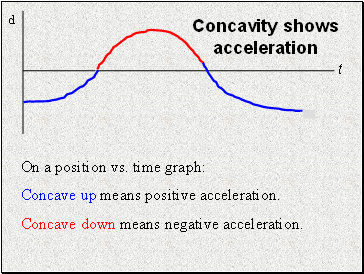Concavity shows acceleration

On a position vs. time graph:

Concave up means positive acceleration.

Concave down means negative acceleration.

Slide 16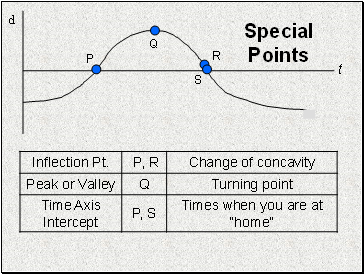Special Points

P

Q

R

S

Slide 17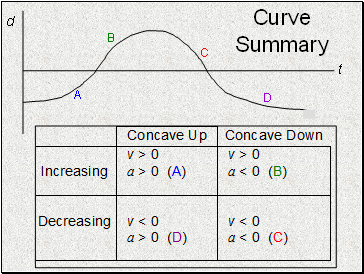Curve Summary

A

B

C

D

Slide 18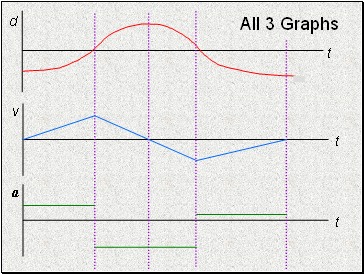All 3 Graphs

v

t

a

t

Slide 19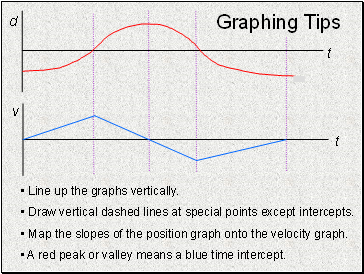Graphing Tips

Line up the graphs vertically.

Draw vertical dashed lines at special points except intercepts.

Map the slopes of the position graph onto the velocity graph.

A red peak or valley means a blue time intercept.

Slide 20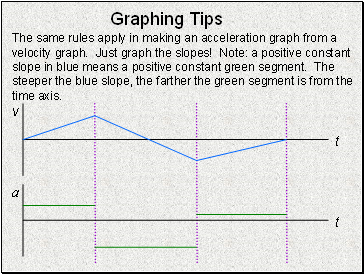## Graphing Tips

The same rules apply in making an acceleration graph from a velocity graph. Just graph the slopes! Note: a positive constant slope in blue means a positive constant green segment. The steeper the blue slope, the farther the green segment is from the time axis.

Go to page:
1  2  3